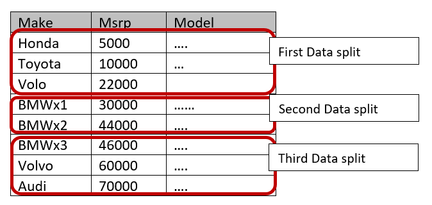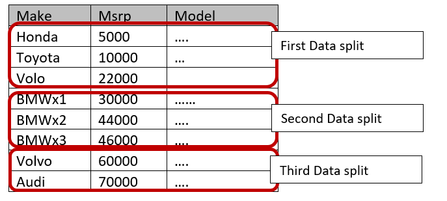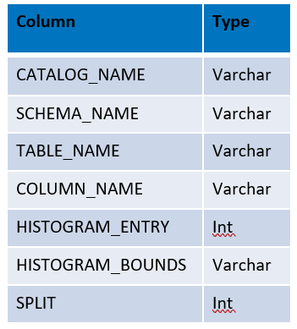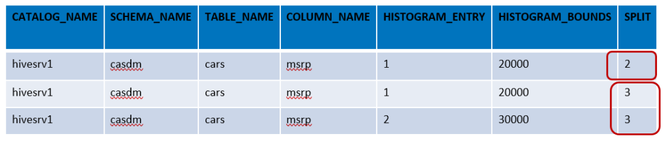## CAS load from Cloud Data Exchange(CDE)

Started ‎06-13-2019 by
Modified ‎06-13-2019 by
Views 1,763

With SAS Viya 3.4 18W38 release, Viya user have a new Data Connector to access and load data from Cloud Data Exchange server to CAS. Cloud Data Exchange (CDE) is a capability to securely access customer’s on-premise data (behind a firewall) from SAS Viya 3.4 application hosted at a public or private cloud environment. This post is about data load from CDE to CAS.

Once the data source services are defined on Data Agent Server, CAS can be loaded from CDE either using Serial or Parallel data load method.

## CDE Serial Data Load to CAS:

The serial data load method transfers data from data agent server to CAS via CAS controller server using single process thread. The CAS controller divides and distribute data blocks amongst the CAS worker nodes in the round-robin fashion.

The following animation describes the serial data load from CDE to CAS:

The following code example describes the serial data load from CDE to CAS. It opens a CAS session and defines a session CASLIB with “clouddex” source type. The CASLIB is using conopts=”DSN=hiveserv1”, a data service defined on data agent server to access Hadoop/Hive tables. The PROC CAS is loading a hive table to CAS through CDE. The Hive table is accessed through CDE using a secured connection between SAS Viya and Data Agent Server.

```CAS mySession  SESSOPTS=( CASLIB=casuser TIMEOUT=99 LOCALE="en_US" metrics=true);

/* Create a Session based Hive_CDE CASLIB */
proc cas;
session mySession;
datasource={srcType="clouddex"
dataAgentName="dagentsrv-shared01-default",
schema="CASDM",
conopts="dsn=hivesrv1"
};
run;
quit;

/** Serial data Load to CAS */
proc cas;
session mySession;
casout={ casLib="hive_cde" , name="cars_srl" } ;
run;
quit;
```

## CDE Parallel Data Load to CAS:

The Following animation describes the parallel data load from CDE to CAS. Two CAS worker nodes read data from Data Agent Server and further distribute the data to rest of non-reader CAS Worker nodes.

### The data split for CAS Worker Nodes

The CAS controller prepares the data split subquery to fetch part of data at reader CAS worker nodes. CDE Data Connector has its own formula to split the data compare to using mod() function on the first numeric column of the data table. There are two ways to split the data for reader CAS Worker nodes.

• Using auto Split with useMinMaxToSplit= option

#### Using Auto Split

The auto split data fetch at CAS is initiated by including numReadNodes > 1, useMinMaxToSplit=TRUE and useMetaTable=FALSE options in load statement. The load statement can also have optional parameters like data split column and data split range. When the split column name is not provided, the first numeric column from the source table is selected. When the split range is not provided, it is calculated on the split column using formula Split Range= ((Max – Min) / numReadNodes).

Each assigned CAS Worker Node will fetch the data by the value of the split range, starting from the minimum value of the split column.

Let’s look at an example with sample data sets to see how the auto split works. Let’s first look at data split with split column and split range. In the following example the source data table (car) has 3 columns make, msrp and model. The load statement includes ‘msrp’ as the split column and the split range of 20000. The 3 reader nodes are being used for data load. Based on given parameters, the CAS will create three data splits with the split range of 20000 starting from min value of ‘msrp’ column.

```…..
useMetaTable=FALSE,
useMinMaxToSplit = TRUE,
splitColumn="msrp",
splitRange = 20000 }
….
```Select any image to see a larger version.
Mobile users: To view the images, select the "Full" version at the bottom of the page.

The following formula is used for data split and to fetch part of data at reader worker nodes.

First Data Split: Inner bound = Min (5000) = 5000 and less Outer bound = Min (5000) + Split Range (20000) = 25000 SQL = “select * from cars where msrp <25000 or msrp is NULL”

Second Data Split: Inner bound = First data split outer bound (25000) = 25000 Outer bound = First data split outer bound (25000) + Split Range (20000) = 45000 SQL = “select * from cars where msrp >= 25000 and msrp < 45000”

Third Data Split: Inner bound = Second data split outer bound (45000) = 45000 Outer bound = Grater than Second data split outer bound (45000) and all = >45000 SQL = “select * from cars where msrp > 45000 “

Now, let’s see how the auto data split works when there is no split column and split range defined in the load statement. In the following example, the ‘msrp’ is selected for the split column since it’s the first numeric column in the data table. The split range is being calculated on msrp column using formula (max-min)/ numReadNodes. The CAS creates three data splits with the calculated split range starting from min value of ‘msrp’ column.

```…..
useMetaTable=FALSE,
useMinMaxToSplit = TRUE  }
….
```The following formula is used for data split and to fetch part of data at reader worker nodes.

Split Range = (Max (70000) – Min (5000) / 3) = 21666

First Data Split: Inner bound = Min (5000) = 5000 and less Outer bound = Min (5000) + Split Range (21666) = 26666 SQL = “select * from cars where msrp <26666 or msrp is NULL”

Second Data Split: Inner bound = First data split outer bound (26666) = 26666 Outer bound = First data split outer bound (26666) + Split Range (21666) = 48332 SQL = “select * from cars where msrp >= 26666 and msrp < 48332”

Third Data Split: Inner bound = Second data split outer bound (48332) = 48332 Outer bound = Grater than Second data split outer bound (48332) and all = >48332 SQL = “select * from cars where msrp > 48332 “

The following code example describes the parallel data load from CDE to CAS using “Auto data split” mechanism with split column and range.

```…..
proc cas;
session mySession;
useMetaTable=FALSE,
useMinMaxToSplit = TRUE,
splitColumn="msrp",
splitRange = 20000,
traceFile="/tmp/cdetrace.log",
traceFlags="SQL"
}
casout={ casLib="hive_cde" , name="cars_splt" } ;
run;
quit;
….
```

With trace file option in data load statement, you can generate a trace log on each CAS worker nodes. You can look at the trace log to verify the SQL submitted from reader CAS worker node to the Data Agent Server. The following is the extract from the trace file generated from the data load statement.

Note: Please don’t compare the Split range listed in the following log with the earlier example. This log is based on data present in cars table.

```Extract from /tmp/cdetrace.log at SASCAS01 server:
[cloud-user@intcas01 tmp]\$ egrep 'Sql=' /tmp/cdetrace.log
In:        StatementHandle=0x7f7320f0a6e0, Sql=`SELECT * FROM "CASDM"."cars" WHERE 0=1`, SqlL=38
In:        StatementHandle=0x7f7320f0a6e0, Sql=`SELECT MIN(msrp), MAX(msrp ) FROM "CASDM"."cars" WHERE msrp IS NOT NULL `, SqlL=72

Extract from /tmp/cdetrace.log at SASCAS02 server:
[cloud-user@intcas02 tmp]\$ egrep 'Sql=' /tmp/cdetrace.log
In:        StatementHandle=0x7ff178144780, Sql=`SELECT * FROM "CASDM"."cars" WHERE  ( "msrp" < 30280 OR "msrp" IS NULL ) `, SqlL=73

Extract from /tmp/cdetrace.log at SASCAS03 server:
[cloud-user@intcas03 ~]\$  egrep 'Sql=' /tmp/cdetrace.log
In:        StatementHandle=0x7fa210026780, Sql=`SELECT * FROM "CASDM"."cars" WHERE  ( "msrp" >= 30280 AND msrp < 50280 ) `, SqlL=73

Extract from /tmp/cdetrace.log at SASCAS04 server:
[cloud-user@intcas04 ~]\$  egrep 'Sql=' /tmp/cdetrace.log
In:        StatementHandle=0x7f84ac086700, Sql=`SELECT * FROM "CASDM"."cars" WHERE  ( "msrp" >= 50280 ) `, SqlL=56
```

To use a metadata-based parallel load, a user must create and populate a metadata table with following structure at the source database. The Metadata table stores information about how to split the data for a given data table. A metadata based parallel (multinode) load is initiated when numReadNodes > 1, metaCatalog=, metaSchema=, metaTable=, and useMetaTable= parameters included in load statement. By default, the value for useMetaTable= is TRUE. Metadata varchar column max length is 256.The column COLUMN_NAME defines the split column from the data table. The column SPLIT defines the number of concurrent data splits to be fetched by multiple reader nodes. For a given SPLIT number, there are as many entries (HISTOGRAM_ENTRY) as (SPLIT - 1) of splits with a bound value (HISTOGRAM_BOUNDS) defined.

Let’s look at an example of metadata based parallel load. The following metadata describes the configuration to use when ‘cars’ table is parallel loaded to CAS using ‘cdemeta’ table. In this example, two ways of data splitting are configured for source table(cars).When numReadNodes=2 in load statement, the configuration of SPLIT=2 will be used for 2 reader nodes to fetch data simultaneously. The first node fetches data where msrp < 20000 and all the NULL value entries. The second node fetches data where msrp >= 20000.

When numReadNodes=3 in load statement, the configuration of SPLIT=3 will be used for 3 reader nodes to fetch data simultaneously. The first node fetches data where msrp < 20000 and all the NULL value entries. The second node fetches data where msrp >= 20000 and msrp < 30000. The third node fetches the data where mspr >= 30000.

The following code example describes the parallel data load from CDE to CAS using a metadata table(cdemeta).

```…..
proc cas;
session mySession;
metaCatalog="hivesrv1",
metaSchema="CASDM",
metaTable="cdemeta
traceFile="/tmp/cdetrace.log",
traceFlags="SQL"
}
casout={ casLib="hive_cde" , name="cars_cdemeta"  } ;
run;
quit;
….
```

The default value ‘cdemeta’ is considered when no value is defined for metaTable= parameter in load statement. If metadata table not found in the source environment, load statement fails.

With trace file option in data load statement, you can generate a trace log on each CAS worker nodes. You can look at the trace log to verify the SQL submitted from reader CAS worker node to the Data Agent Server. The following is the extract from the trace file generated from the data load statement.

```Extract from /tmp/cdetrace.log at SASCAS01 server:
[cloud-user@intcas01 tmp]\$ egrep 'Sql=' /tmp/cdetrace.log
In:        StatementHandle=0x7fe74bddecc0, Sql=`SELECT CATALOG_NAME, SCHEMA_NAME, TABLE_NAME, COLUMN_NAME, HISTOGRAM_ENTRY, HISTOGRAM_BOUNDS, SPLIT FROM "hivesrv1"."CASDM"."cdemeta" WHERE UPCASE(SCHEMA_NAME)=UPCASE('CASDM') AND UPCASE(TABLE_NAME)=UPCASE('cars') ORDER BY SPLIT, HISTOGRAM_ENTRY`, SqlL=245

In:        StatementHandle=0x7fe74bddecc0, Sql=`SELECT * FROM "CASDM"."cars" WHERE 0=1`, SqlL=38

Extract from /tmp/cdetrace.log at SASCAS02 server:
[cloud-user@intcas02 tmp]\$ egrep 'Sql=' /tmp/cdetrace.log

In:        StatementHandle=0x7f16dc043ec0, Sql=`SELECT * FROM "CASDM"."cars" WHERE  ( "msrp" < 20000 OR "msrp" IS NULL ) `, SqlL=73

Extract from /tmp/cdetrace.log at SASCAS03 server:
[cloud-user@intcas03 ~]\$  egrep 'Sql=' /tmp/cdetrace.log
In:        StatementHandle=0x7fe4180982e0, Sql=`SELECT * FROM "CASDM"."cars" WHERE  ( "msrp" >= 20000 AND msrp < 30000 ) `, SqlL=73

Extract from /tmp/cdetrace.log at SASCAS04 server:
[cloud-user@intcas04 ~]\$  egrep 'Sql=' /tmp/cdetrace.log

In:        StatementHandle=0x7fa08814b700, Sql=`SELECT * FROM "CASDM"."cars" WHERE  ( "msrp" >= 30000 ) `, SqlL=56
```

When using metadata for parallel data load make sure to populate cdemeta table with latest histogram data. Choose the split column name based on data cardinality. This is a tedious task to populate the ‘cdemeta’ table, but it’s one of the feature with CDE data connector for parallel data load.

### Resources:

Version history
Last update:
‎06-13-2019 11:56 AM
Updated by:Contributors
Article Labels
Article Tags